# Forced Convective Heat Transfer - 6 Notes | Study Heat Transfer - Mechanical Engineering

## Mechanical Engineering: Forced Convective Heat Transfer - 6 Notes | Study Heat Transfer - Mechanical Engineering

The document Forced Convective Heat Transfer - 6 Notes | Study Heat Transfer - Mechanical Engineering is a part of the Mechanical Engineering Course Heat Transfer.
All you need of Mechanical Engineering at this link: Mechanical Engineering

4.4.1 Reynolds analogy
Reynolds has taken the following assumptions to find the analogy between heat and momentum transport.

1. Gradients of the dimensionless parameters at the wall are equal.
2. The diffusivity terms are equal. That is

Thus if we use the above assumptions along with the eq.4.32 and 4.33,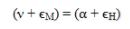Thus if we use the above assumptions along with the eq.4.32 and 4.33,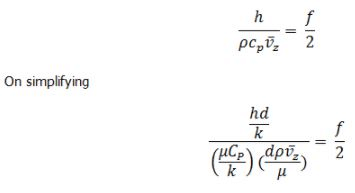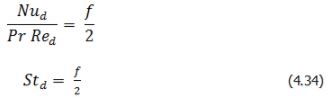Equation 4.34 is known as Reynolds’s analogy.
The above relation may also be written in terms of the Darcy’s friction factor (fD) instead of fanning friction factor (fD = 4f)
Where Stanton number (St) is defined as,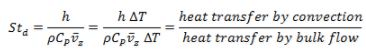The advantage of the analogy lies in that the h may not be available for certain geometries/situations however, for which f value may be available as it is easier to perform momentum transport experiments and then to calculate the f. Thus by using the eq.4.34 the h may be found out without involving into the exhaustive and difficult heat transfer experiments.

4.4.2 The Chilton-Colburn analogy
The Reynolds analogy does not always give satisfactory results. Thus, Chilton and Colburn experimentally modified the Reynolds’ analogy. The empirically modified Reynolds’ analogy is known as Chilton-Colburn analogy and is given by eq.4.35,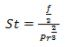(4.35 a)

or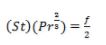(4.35 b)

It can be noted that for unit Prandtl number the Chilton-Colburn analogy becomes Reynolds analogy.

In the turbulent core the transport is mainly by eddies and near the wall, that is laminar sub-layer, the transport is by molecular diffusion. Therefore, Prandtl modified the above two analogies using universal velocity profile while driving the analogy (eq. 4.36).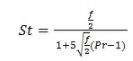(4.36)

4.4.4 The Van Karman analogy

Though Prandtl considered the laminar and turbulent laminar sublayers but did not consider the buffer zone. Thus, Van Karman included the buffer zone into the Prandtl analogy to further improve the analogy.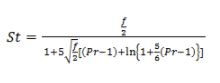(4.37)

The document Forced Convective Heat Transfer - 6 Notes | Study Heat Transfer - Mechanical Engineering is a part of the Mechanical Engineering Course Heat Transfer.
All you need of Mechanical Engineering at this link: Mechanical EngineeringUse Code STAYHOME200 and get INR 200 additional OFF

## Heat Transfer

58 videos|70 docs|85 tests

Track your progress, build streaks, highlight & save important lessons and more!

,

,

,

,

,

,

,

,

,

,

,

,

,

,

,

,

,

,

,

,

,

;